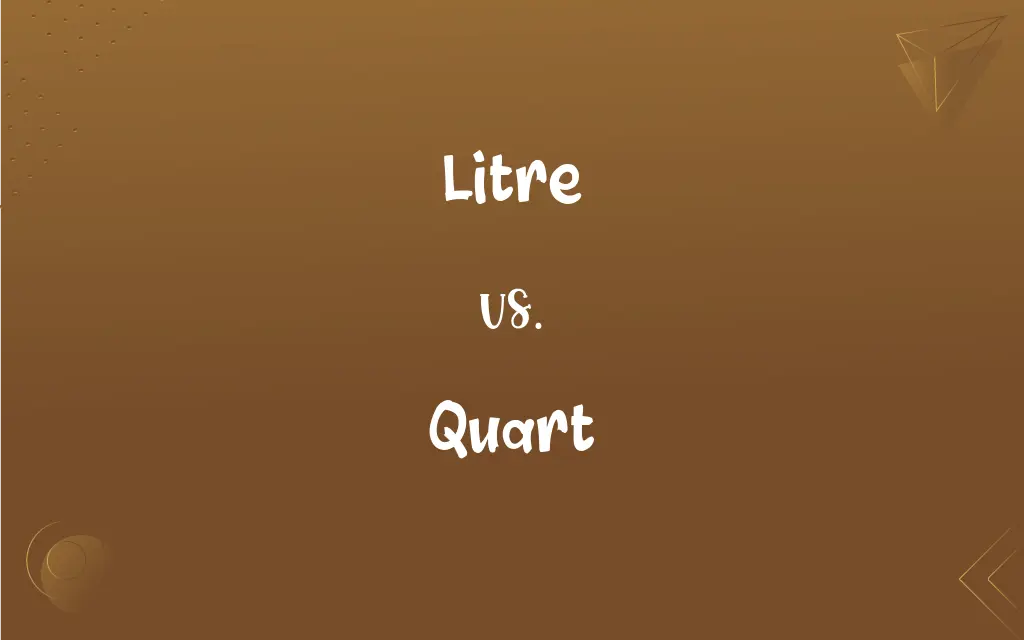# Litre vs. Quart## Litre and Quart Definitions

#### Litre

Variant of liter.

#### Quart

A unit of volume or capacity in the US Customary System, used in liquid measure, equal to 1/4 gallon or 32 ounces (0.946 liter).

#### Litre

The metric unit of fluid measure, equal to one cubic decimetre. Symbols: l, L, ℓ
You should be able to fill four cups with one litre of water.

#### Quart

A unit of volume or capacity in the US Customary System, used in dry measure, equal to 1/8 peck or 2 pints (1.101 liters).

#### Litre

(informal) A measure of volume equivalent to a litre.

#### Quart

A unit of volume or capacity in the British Imperial System, used in liquid and dry measure, equal to 1.201 US liquid quarts or 1.032 US dry quarts (1.136 liters). See Table at measurement.

Same as Liter.

#### Quart

A container having a capacity of one quart.

#### Litre

A metric unit of capacity equal to the volume of 1 kilogram of pure water at 4 degrees centigrade and 760 mm of mercury (or approximately 1.76 pints)

#### Quart

The contents of such a container.

#### Quart

A unit of liquid capacity equal to two pints; one-fourth (quarter) of a gallon. Equivalent to 1.136 liters in the UK and 0.946 liter (liquid quart) or 1.101 liters (dry quart) in the U.S.

#### Quart

(cards) Four successive cards of the same suit.

#### Quart

(obsolete) A fourth; a quarter; hence, a region of the earth.

#### Quart

The fourth part; a quarter; hence, a region of the earth.
Camber did possess the western quart.

#### Quart

A measure of capacity, both in dry and in liquid measure; the fourth part of a gallon; the eighth part of a peck; two pints.

#### Quart

A vessel or measure containing a quart.

#### Quart

In cards, four successive cards of the same suit. Cf. Tierce, 4.

#### Quart

A United States liquid unit equal to 32 fluid ounces; four quarts equal one gallon